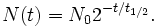SEARCH HOMEMath Central Quandaries & QueriesQuestion from Christine, a parent: How do I set up the exponential decay formula for Carbon 14 with an initial amount of 1500 grams.Hi Christine.

The general formula for exponential decay given a half-life iswhere N(t) is the amount at time t, N0 is the initial amount (at time t=0), and t1/2 is the half life of the substance.

Carbon-14 (14C) is a radioactive substance that has a half-life of about 5730 years. While a biological organism is alive and respiring, it absorbs 14C from the air and has a constant percentage in its tissues. When the organism dies, the amount of 14C gradually diminishes in accordance with the formula above. This makes it possible to measure the percentage of remaining 14C in dead organic matter and determine the approximate time of death.

You can read more about the science of radiocarbon dating here.

Stephen La Rocque.>Math Central is supported by the University of Regina and The Pacific Institute for the Mathematical Sciences.